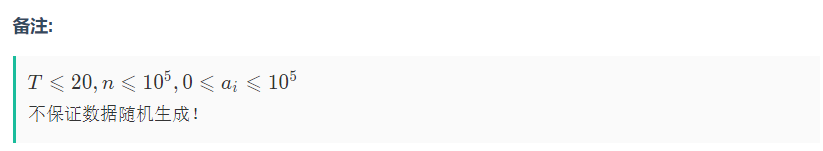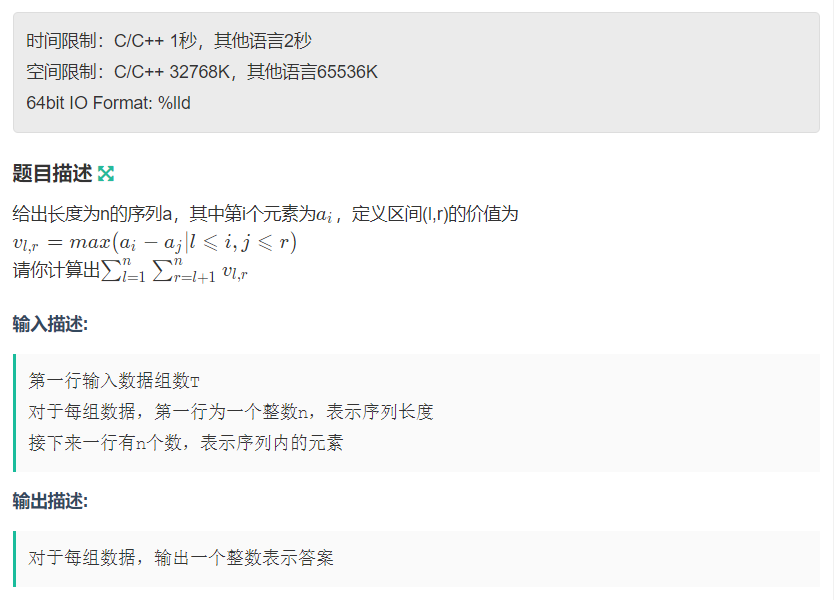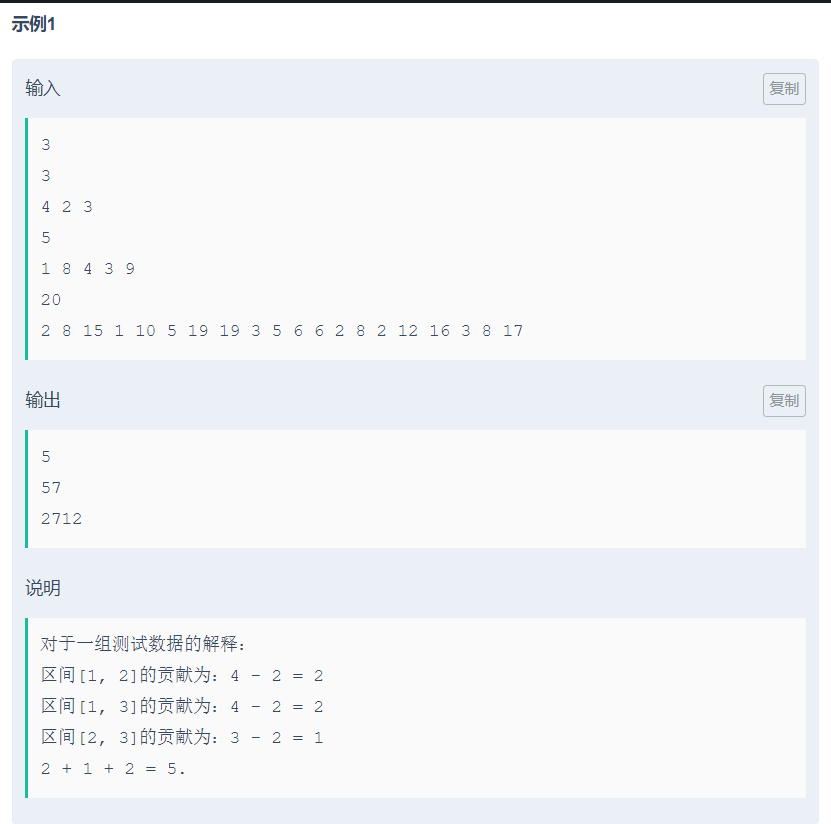## 题目6 5 5 4 8

2 4

### CODE

#include<iostream>
#include<cstdio>
#include<set>
#include<cmath>
#include<cstring>
#include<string>
#include<map>
#include<vector>
#include<queue>
#include<stack>
#include<algorithm>
#define ios ios::sync_with_stdio(0); cin.tie(0); cout.tie(0)
typedef long long ll;
using namespace std;
const int MAXN=1e5+100;
ll l[MAXN],r[MAXN],a[MAXN];
ll fun(ll n){
stack<ll> st1,st2;
memset(l,0,sizeof l);
memset(r,0,sizeof r);
for(ll i=1;i<=n;i++){
while(st1.size()&&a[i]>=a[st1.top()]){
st1.pop();
}
if(st1.empty()) l[i]=1;
else l[i]=st1.top()+1;
st1.push(i);
}
for(ll i=n;i;i--){
while(st2.size()&&a[i]>a[st2.top()]){ //一定注意这里是大于号
st2.pop();
}
if(st2.empty()) r[i]=n;
else r[i]=st2.top()-1;
st2.push(i);
}
ll ans=0;
for(ll i=1;i<=n;i++){
ans+=a[i]*((r[i]-l[i])+(i-l[i])*(r[i]-i));
}
return ans;
}
int main()
{
ios;
ll t;
cin>>t;
while(t--){
ll n; cin>>n;
for(ll i=1;i<=n;i++) cin>>a[i];
ll ans=0;
ans+=fun(n);
for(ll i=1;i<=n;i++) a[i]=-a[i];
ans+=fun(n);
cout<<ans<<'\n';
}
return 0;
}

••